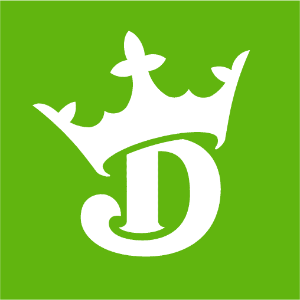DraftKings NFC Championship Odds
+475
4.75 to 149ers
14.8% implied probability

+600
6 to 1Saints
12.2% implied probability

+700
7 to 1Buccaneers
10.6% implied probability

+850
8.5 to 1Cowboys
9.0% implied probability

+1000
10 to 1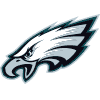Eagles
7.7% implied probability

+1000
10 to 1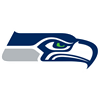Seahawks
7.7% implied probability

+1100
11 to 1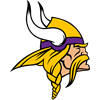Vikings
7.1% implied probability

+1300
13 to 1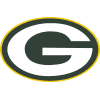Packers
6.1% implied probability

+1600
16 to 1Rams
5.0% implied probability

No changes have been recorded yet.
+2000
20 to 1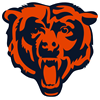Bears
4.1% implied probability

+2200
22 to 1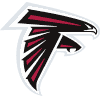Falcons
3.7% implied probability

+2500
25 to 1Cardinals
3.3% implied probability

No changes have been recorded yet.
+3000
30 to 1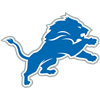Lions
2.7% implied probability

+3000
30 to 1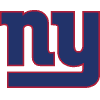Giants
2.7% implied probability

+5000
50 to 1Panthers
1.7% implied probability

+5000
50 to 1Football Team
1.7% implied probability